# Single-Source Shortest Paths – Bellman–Ford AlgorithmGiven a source vertex `s` from a set of vertices `V` in a weighted directed graph where its edge weights `w(u, v)` can be negative, find the shortest path weights `d(s, v)` from source `s` for all vertices `v` present in the graph. If the graph contains a negative-weight cycle, report it.

For example, consider the following graph: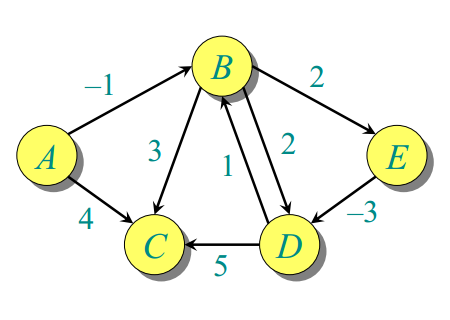Let source vertex = 0,

The distance of vertex 1 from vertex 0 is -1. Its path is [0 —> 1]
The distance of vertex 2 from vertex 0 is 2. Its path is [0 —> 1 —> 2]
The distance of vertex 3 from vertex 0 is -2. Its path is [0 —> 1 —> 4 —> 3]
The distance of vertex 4 from vertex 0 is 1. Its path is [0 —> 1 —> 4]

Practice this problem

The idea is to use the Bellman–Ford algorithm to compute the shortest paths from a single source vertex to all the other vertices in a given weighted digraph. Bellman–Ford algorithm is slower than Dijkstra’s Algorithm, but it can handle negative weights edges in the graph, unlike Dijkstra’s.

If a graph contains a “negative cycle” (i.e., a cycle whose edges sum to a negative value) that is reachable from the source, then there is no shortest path. Any path that has a point on the negative cycle can be made cheaper by one more walk around the negative cycle. Bellman–Ford algorithm can easily detect any negative cycles in the graph.

The algorithm initializes the distance to the source to `0` and all other nodes to `INFINITY`. Then for all edges, if the distance to the destination can be shortened by taking the edge, the distance is updated to the new lower value. At each iteration `i` that the edges are scanned, the algorithm finds all shortest paths of at most length `i` edges. Since the longest possible path without a cycle can be `V-1` edges, the edges must be scanned `V-1` times to ensure that the shortest path has been found for all nodes. A final scan of all the edges is performed, and if any distance is updated, then a path of length `|V|` edges have been found, which can only occur if at least one negative cycle exists in the graph.

Following is the pseudocode for Bellman–Ford as per Wikipedia. The implementation takes a graph, represented as lists of vertices and edges, and fills `distance[]` and `parent[]` with the shortest path (least cost/path) information:

function BellmanFord(list vertices, list edges, vertex source, distance[], parent[])

// Step 1 – initialize the graph. In the beginning, all vertices weight of
// INFINITY and a null parent, except for the source, where the weight is 0

for each vertex v in vertices
distance[v] = INFINITY
parent[v] = NULL

distance[source] = 0
// Step 2 – relax edges repeatedly
for i = 1 to V-1    // V – number of vertices
for each edge (u, v) with weight w
if (distance[u] + w) is less than distance[v]
distance[v] = distance[u] + w
parent[v] = u

// Step 3 – check for negative-weight cycles
for each edge (u, v) with weight w
if (distance[u] + w) is less than distance[v]
return “Graph contains a negative-weight cycle”

return distance[], parent[]

The following slideshow illustrates the working of the Bellman–Ford algorithm. The images are taken from MIT 6.046J/18.401J Introduction to Algorithms (Lecture 18 by Prof. Erik Demaine).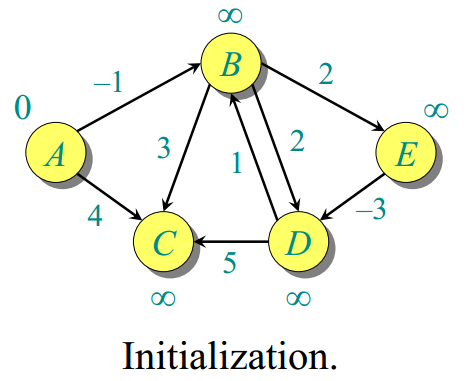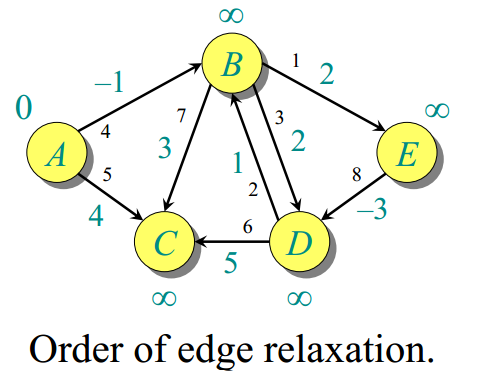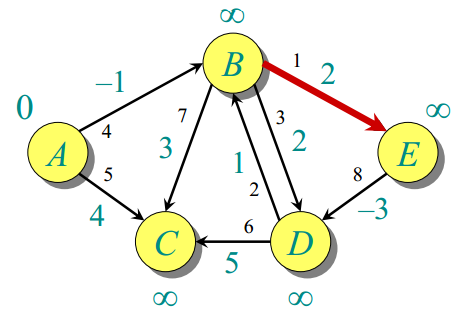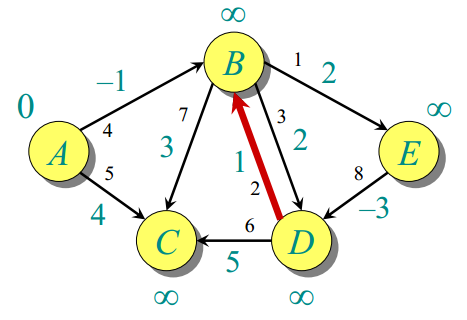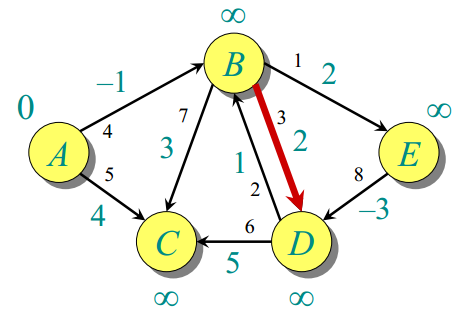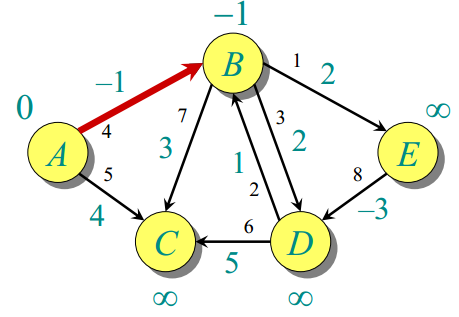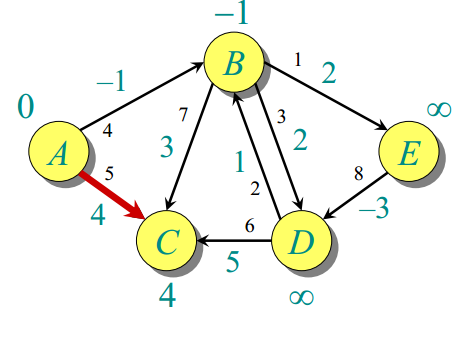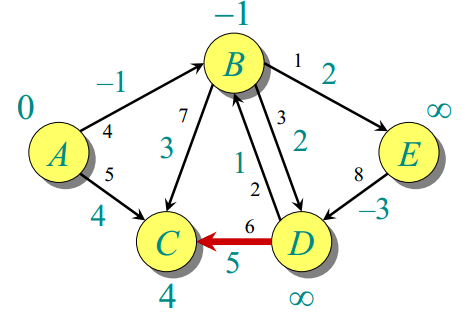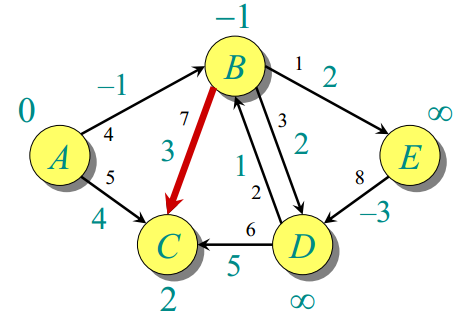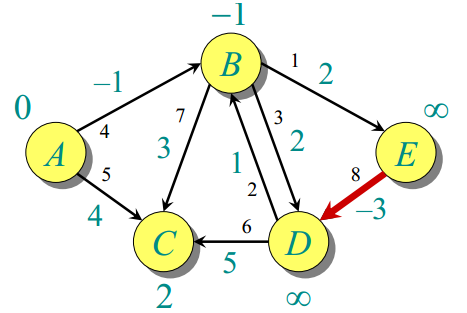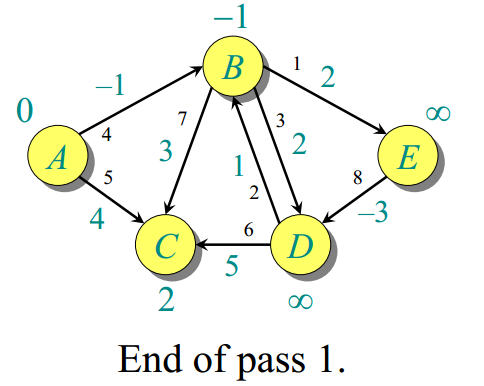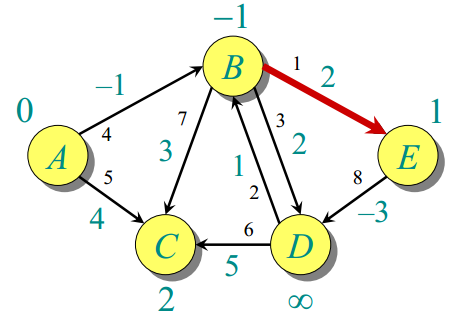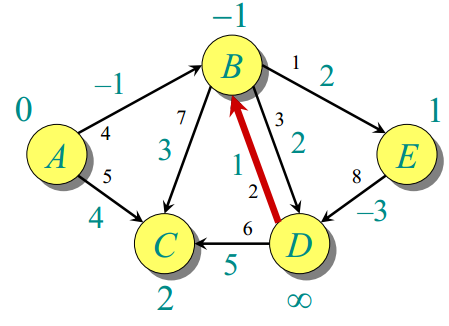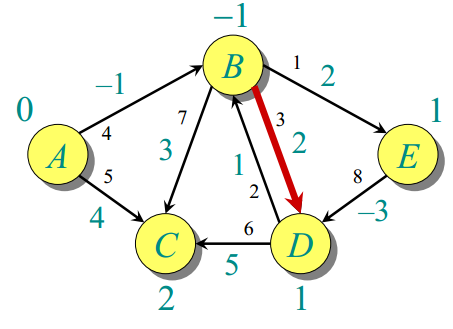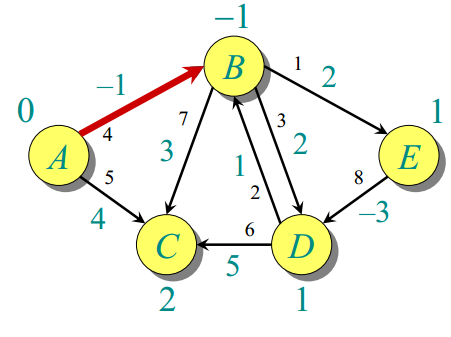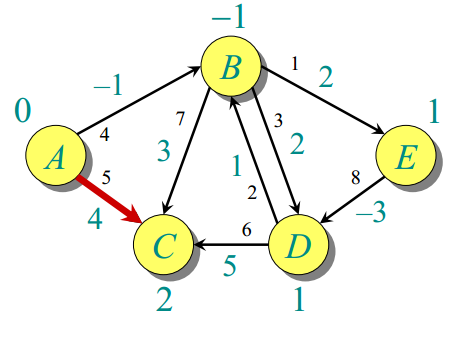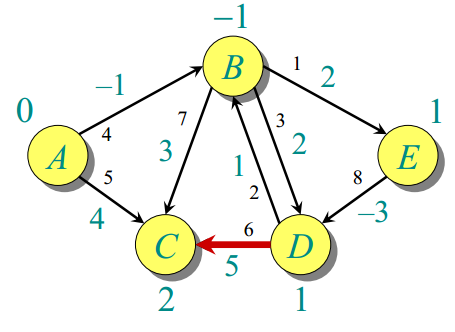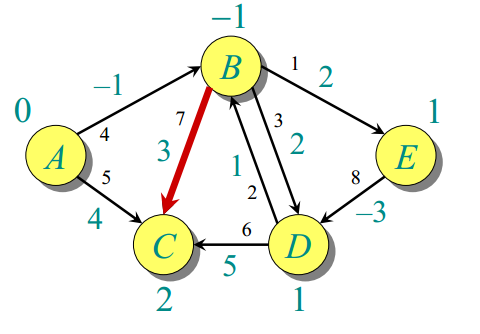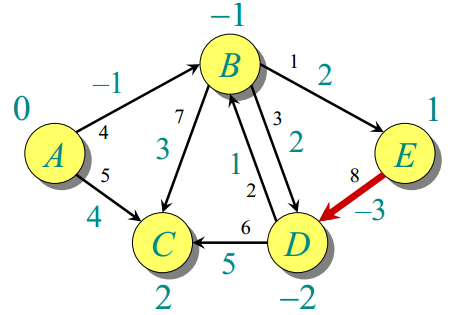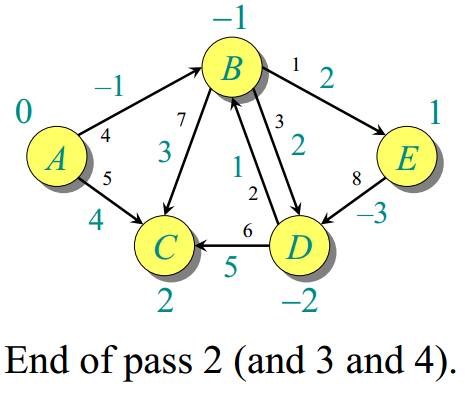The algorithm can be implemented as follows in C++, Java, and Python:

## Python

Output:

The distance of vertex 1 from vertex 0 is -1. Its path is [0, 1]
The distance of vertex 2 from vertex 0 is 2. Its path is [0, 1, 2]
The distance of vertex 3 from vertex 0 is -2. Its path is [0, 1, 4, 3]
The distance of vertex 4 from vertex 0 is 1. Its path is [0, 1, 4]
The distance of vertex 2 from vertex 1 is 3. Its path is [1, 2]
The distance of vertex 3 from vertex 1 is -1. Its path is [1, 4, 3]
The distance of vertex 4 from vertex 1 is 2. Its path is [1, 4]
The distance of vertex 1 from vertex 3 is 1. Its path is [3, 1]
The distance of vertex 2 from vertex 3 is 4. Its path is [3, 1, 2]
The distance of vertex 4 from vertex 3 is 3. Its path is [3, 1, 4]
The distance of vertex 1 from vertex 4 is -2. Its path is [4, 3, 1]
The distance of vertex 2 from vertex 4 is 1. Its path is [4, 3, 1, 2]
The distance of vertex 3 from vertex 4 is -3. Its path is [4, 3]

The time complexity of the Bellman–Ford algorithm is O(V × E), where `V` and `E` are the total number of vertices and edges in the graph, respectively.

References:

Rate this post

Average rating 4.93/5. Vote count: 164

No votes so far! Be the first to rate this post.

We are sorry that this post was not useful for you!

Tell us how we can improve this post?